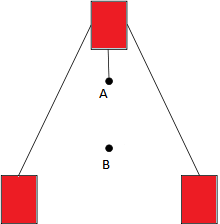# Centre of mass

The centre of mass of a system of particles can be regarded as the mass weighted average location of the constituent particles. When we deal with rigid bodies of continuous distribution of matter we need to replace the summation symbol with an integral symbol.

Consider a body be made up of bid number of particles which mass is equal to the total mass of all the particles. When such a body undergoes a translational motion the move is produced in each and every particle of the body with respect to their original position

• If this body is executing motion under the effect of some external forces acting on it then it will be get that there’s a point in the system , where if all mass of the system is supposed to be concentrated and the nature the motion executed by the system remains unaltered when force acts on the system is directly applied to this point. Such a point of the system is called centre of mass of the system.
• Hence for any system Centre of mass is the point where whole mass of the system can be supposed to be concentrated and motion of the system can be defined in terms of the centre of mass.
•### Centre of Mass Formula

$$x_{com} = \frac{\sum_{n}^{i=0}m_ix_i}{M}$$ $$y_{com} = \frac{\sum_{n}^{i=0}m_iy_i}{M}$$ $$z_{com} = \frac{\sum_{n}^{i=0}m_iz_i}{M}$$

To find the center of mass of an extended object like a rod, then consider a differential mass and its position and then integrate it over the entire length.

$$x_{com} = \frac{\int xdm}{M}$$ $$y_{com} = \frac{\int ydm}{M}$$ $$z_{com} = \frac{\int zdm}{M}$$

Where,

xcom, ycom and zcom = Center of mass along x, y, and z-axis,

M = The total mass of system,

n = Number of objects,

mi = Mass of the ith object and

xi = Distance from the x-axis of ith object

yi = Distance from the y-axis of ith object

zi = Distance from the z-axis of ith object